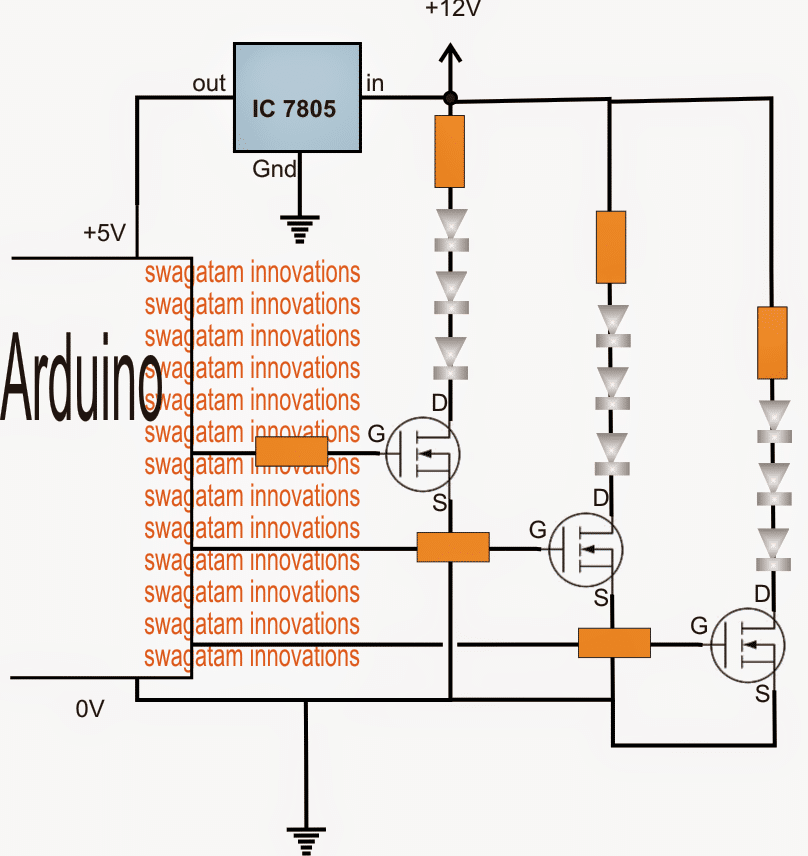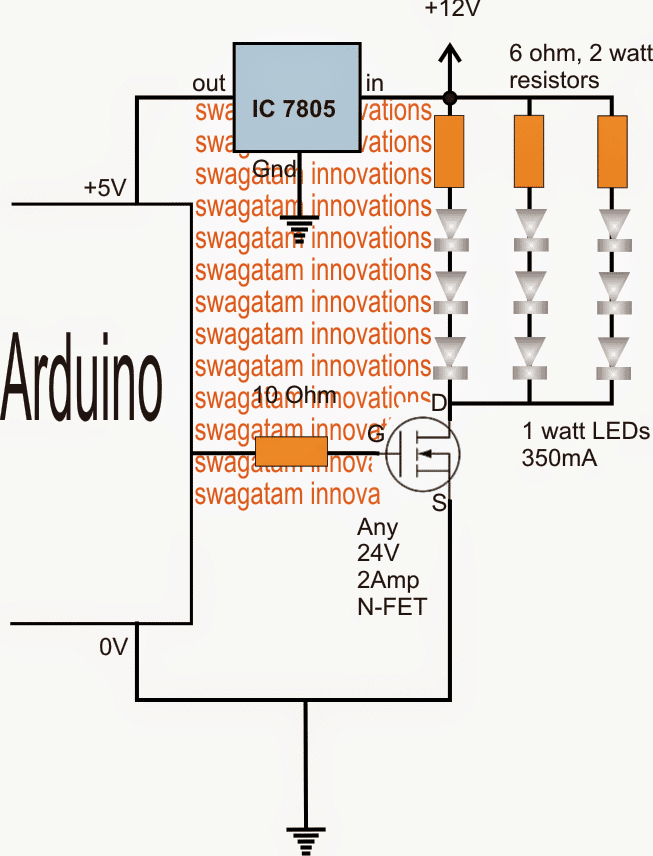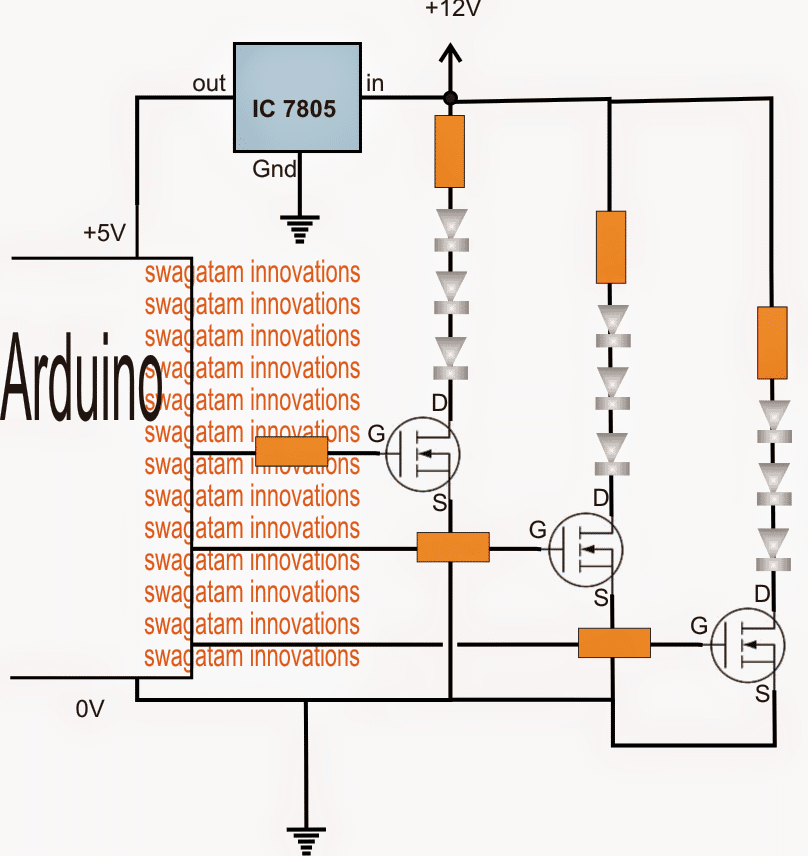Home » Audio Projects » How to Drive High Watt LEDs with ArduinoHow to Drive High Watt LEDs with Arduino

The post explains the method of incorporating high watt LEDs with Arduino through external high voltage supplies. The question was put forth by Mr. Cole.

The Circuit Question

I stumbled upon your blog and I love it! So much great information and great ideas Right now I am trying to figure out exactly how to separately control several 1 watt leds from the arduino ,

I understand c language and have no problem with the arduino, I just don't really understand how to run higher voltage through the arduino since it puts out 5v I understand a little about mosfets and planned on using logic level mosfets to control these leds..

they will only be flashing a few times a minute for about 30 minutes a day..do you see any problem running them through mosfets? Will I need 9 mosfets to separately control 9 leds?

Do I need resistors as well or do the mosfets make up for that?

Any help would be much appreciated! Thanks again!

Cole

The Circuit Solution:

For controlling 9nos of 1 watt LEDs together through an Arduino, the following simple set up may be incorporated through a 12V external supply:For controlling single LEDs or multiple LEDs from separate Arduino outputs, individual mosfets may be required as given below:The LED resistors may be calculated using the following formula:

R = (U - LEDfwdV)/LED Current

where U is the supply voltage

LEDfwdV is the LED forward operating voltage of the particular series

LED current is the ampere rating specs of the LEDs used

Therefore here U = 12V

LEDfwdV = 3.3V x 3 = 9.9V since 3nos are there in each series and 3.3V being the forward voltage spec of each LED

LED current = 350mA, let's take it 300mA or 0.3Amp to keep things cooler.

Substituting these in the formula:

R = (U - LEDfwdV)/LED Current

= 12 - 9.9/0.3

= 7 ohm

watts may be calculated as

Watts = LEDfwdV x LED current = 9.9 x 0.3 = 2.97 watts or 3 watts

SHARING IS CARING!1.